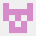강의로 돌아가기허용되지 않는 시스템 호출 뜨는 이유?

import numpy as np
def solution(bridgelength, weight, truckweights):
time =0
bridge=list(np.zeros(bridgelength,dtype=int))
merge
bridge = bridge+truckweights
while sum(merge
bridge) != 0:
if sum(mergebridge[:bridgelength+1]) < weight:
del mergebridge
merge
bridge.append(0)
else:
del mergebridge
merge
bridge.insert(bridge_length-1,0)

``````    time += 1

return time
``````
작성중인 코드―solution.py
``````1
2
3
4
5
6
7
8
9
10
11
12
13
14
15
16
import numpy as np
def solution(bridge_length, weight, truck_weights):
time =0
bridge=list(np.zeros(bridge_length,dtype=int))
merge_bridge = bridge+truck_weights
while sum(merge_bridge) != 0:
if sum(merge_bridge[:bridge_length+1]) < weight:
del merge_bridge
merge_bridge.append(0)
else:
del merge_bridge
merge_bridge.insert(bridge_length-1,0)

time += 1

return time
``````
1 개의 답변https://www.welcomekakao.com/learn/questions/3577

numpy 가 표준 라이브러리가 아니어서 그렇습니다.